## Example Questions

← Previous 1 3 4 5 6

### Example Question #1 : How To Find The Equation Of A Perpendicular Line

The equation of line p is  y= 1/4x +6.  If line k contains the point (3,5) and is perpendicular to line p, find the equation of line k.

y = 1/4x + 17

y = 3x + 5

y = -4x + 17

y = 4x - 17

y = -4x + 17

Explanation:

Using the slope intercept formula, we can see the slope of line p is ¼. Since line k is perpendicular to line p it must have a slope that is the negative reciprocal. (-4/1) If we set up the formula y=mx+b, using the given point and a slope of (-4), we can solve for our b or y-intercept. In this case it would be 17.

### Example Question #2 : How To Find The Equation Of A Perpendicular Line

In the xy-coordinate plane, a line A contains the points (0,0) and (3,1). If the line B is perpendicular to A at (3,1), what is the equation of the line?

y = -1/3x + 10

y = -3x + 1

y = -3x + 10

y = 1/3x + 1

y = 3x + 1

y = -3x + 10

Explanation:

First, you need to obtain the equation of the first line, A. Its slope is given by:

(y2 - y1) / (x2 - x1) = (1 - 0) / (3 - 0) = 1/3 = slope of A.

Remember that the slope of a perpendicular line to a given line is -1 times the inverse of its slope. Thus the slope of B:

(-1) x 1 / (1/3) = -3

Thus with y = mx + b, m = -3. Now the line must include (3,1). Thus:

with y = -3x + b:

1 = -3(3) + b;

1 = -9 + b; add 9 to both sides:

10 = b

### Example Question #3 : How To Find The Equation Of A Perpendicular Line

What line is perpendicular to the line 2x + 3y = 6 through (4, 1)?

–3x + 2y = –10

–2x + 3y = 5

–x + 4y = 8

3x + 2y = 10

2x + 3y = –5

–3x + 2y = –10

Explanation:

The given equation is in standard form, so it must be converted to slope-intercept form: y = mx + b to discover the slope is –2/3. To be perpendicular the new slope must be 3/2 (opposite reciprocal of the old slope). Using the new slope and the given point we can substitute these values back into the slope-intercept form to find the new intercept, –5. In slope-intercept form the new equation is y = 3/2x – 5. The correct answer is this equation converted to standard form.

### Example Question #4 : How To Find The Equation Of A Perpendicular Line

The endpoints of line segment AB are located at (5, –2) and (–3, 10). What is the equation of the line that is the perpendicular bisector of AB?

2x – 3y = –10

3x – 4y = –4

3x – 4y = –13

2x – 3y = –20

2x + 3y = 14

2x – 3y = –10

Explanation:

We are asked to find the equation of the line that is the perpendicular bisector of AB. If we find a point that the line passes through as well as its slope, we can determine its equation. In order for the line to bisect AB, it must pass through the midpoint of AB. Thus, one point on the line is the midpoint of the AB. We can use the midpoint formula to determine the midpoint of AB with endpoints (5, –2) and (–3, 10).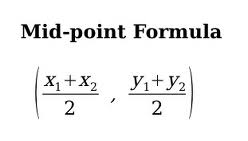The x-coordinate of the midpoint is located at (5 + –3)/2 = 1.

The y-coordinate of the midpoint is located at (–2 + 10)/2 = 4.

Thus, the midpoint of AB is (1, 4).

So, we know that the line passes through (1,4). Now, we can use the fact that the line is perpendicular to AB to find its slope. The product of the slopes of two line segments that are perpendicular is equal to –1. In other words, if we multiply the slope of the line by the slope of AB, we will get –1.

We can use the slope formula to find the slope of AB.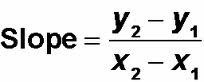slope of AB = (10 – (–2))/(–3 – 5) = 12/–8 = –3/2.

Since the slope of the line multiplied by –3/2 must equal –1, we can write the following:

(slope of the line)(–3/2) = –1

If we multiply both sides by –2/3, we will find the slope of the line.

The slope of the line = (–1)(–2/3) = 2/3.

Thus, the line passes through the ponit (1, 4) and has a slope of 2/3.

We will now use point-slope form to determine the line's equation. Let's let m represent the slope and (x1, y1) represent a ponit on the line.

y – y = m(x – x1)

y – 4 = (2/3)(x – 1)

Multiply both sides by 3 to get rid of the fraction.

3(y – 4) = 2(x – 1)

Distribute both sides.

3y – 12 = 2x – 2

Subtract 3y from both sides.

–12= 2x – 3y – 2

–10 = 2x – 3y.

The equation of the line is 2x – 3y = –10.

The answer is 2x – 3y = –10.

### Example Question #5 : How To Find The Equation Of A Perpendicular Line

A line passes through (2, 8) and (4, 15). What is a possible equation for a line perpendicular to this one?

y = (–7/2)x + 4

y = (–2/7)x + 4

y = (2/7)x + 4

y = (8/3)x + 4

y = (–2/7)x + 4

Explanation:

Remember, perpendicular lines have opposite-reciprocal slopes; therefore, let's first find the slope of our line. That is found by the equation: rise/run or y2 – y1/x2 – x1

Substituting in our values: (15 – 8)/(4 – 2) = 7/2

The perpendicular slope is therefore –2/7.

Since ANY perpendicular line will intersect with this line at some point. We merely need to choose the answer that has a line with slope –2/7. Following the slope intercept form (y = mx + b), we know that the coefficient of x will give us this; therefore our answer is: y = (–2/7)x + 4

### Example Question #1 : How To Find The Equation Of A Perpendicular Line

Line p is given by the equation y = –x + 4. Which of the following equations describes a line that is perpendicular to p?

y = x + 4

x + y = –4

y = –x – 4

x + y = 4

y = –4

y = x + 4

Explanation:

The equation of line p is given in the form y = mx + b, where m is the slope and b is the y-intercept. Because the equation is y = –x + 4, the slope is m = –1.

If two lines perpendicular, then the product of their slopes is equal to –1. Thus, if we call n the slope of a line perpendicular to line p, then the following equation is true:

m(n) = –1

Because the slope of line p is –1, we can write (–1)n = –1. If we divide both sides by –1, then n = 1. In short, the slope of a line perpendicular to line p must equal 1. We are looking for the equation of a line whose slope equals 1.

Let's examine the answer choices. The equation y = –x – 4 is in the form y = mx + b (which is called point-slope form), so its slope is –1, not 1. Thus, we can eliminate this choice.

Next, let's look at the line x + y = 4. This line is in the form Ax + By = C, where A, B, and C are constants. When a line is in this form, its slope is equal to –A/B. Therefore, the slope of this line is equal to –1/1 = –1, which isn't 1. So we can eliminate x + y = 4. Simiarly, we can eliminate the line x + y = –4.

The line y = –4 is a horizontal line, so its slope is 0, which isn't 1.

The answer is the line y = x + 4, because it is the only line with a slope of 1.

The answer is y = x + 4.

### Example Question #1 : How To Find The Equation Of A Perpendicular Line

Give the equation of a line perpendicular to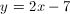.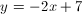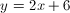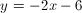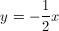Explanation:

The slope of a perpendicular line is the opposite reciprocal, therefore we are looking for a line with a slope of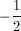.is the only answer choice that satisfies this criteria.

### Example Question #1 : How To Find The Equation Of A Perpendicular Line

Find the equation of the line that is perpendicular to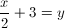and passes through (5, 6).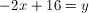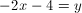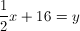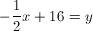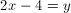Explanation:

We know that the slope of the original line is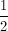Thus the slope of the perpendicular line is the negative reciprocal of, or –2.

Then we plug the slope and point (5, 6) into the form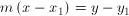, which yields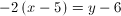When we simplify this, we arrive at### Example Question #1 : How To Find The Equation Of A Perpendicular Line

What line is perpendicular to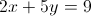through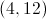?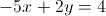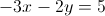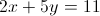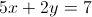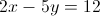Explanation:

We need to find the slope of the given equation by converting it to the slope intercept form: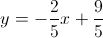The slope is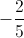and the perpendicular slope would be the opposite reciprocal, or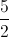The new equation is of the form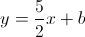and we can use the pointto calculate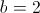. The next step is to convert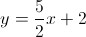into the standard form of.

### Example Question #1 : How To Find The Equation Of A Perpendicular Line

What line is perpendicular to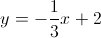and passes through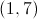?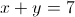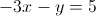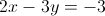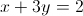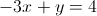Explanation:

Perpendicular slopes are opposite reciprocals.  The original slope is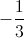so the new perdendicular slope is 3.

We plug the pointand the slope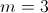into the point-slope form of the equation: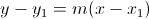to get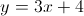or in standard form.

← Previous 1 3 4 5 6

### All SAT Math Resources